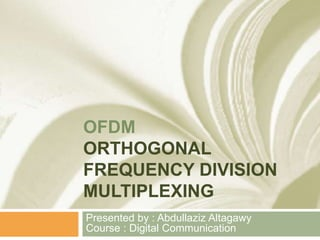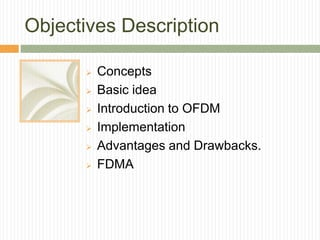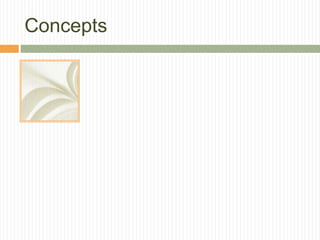Diese Präsentation wurde erfolgreich gemeldet.

# OFDM Orthogonal Frequency Division Multiplexing

Anzeige
Anzeige
Anzeige
Anzeige
Anzeige
Anzeige
Anzeige
Anzeige
Anzeige
Anzeige
Anzeige×

1 von 55 Anzeige

# OFDM Orthogonal Frequency Division Multiplexing

OFDM allows tightly packed carriers to convey information orthogonally and with high bandwidth efficiency
Objectives Description:
Concepts
Basic idea
Introduction to OFDM
Implementation
FDMA

OFDM allows tightly packed carriers to convey information orthogonally and with high bandwidth efficiency
Objectives Description:
Concepts
Basic idea
Introduction to OFDM
Implementation
FDMA

Anzeige
Anzeige

### OFDM Orthogonal Frequency Division Multiplexing

1. 1. OFDM ORTHOGONAL FREQUENCY DIVISION MULTIPLEXING Presented by : Abdullaziz Altagawy Course : Digital Communication
2. 2. Objectives Description  Concepts  Basic idea  Introduction to OFDM  Implementation  Advantages and Drawbacks.  FDMA
3. 3. Concepts
4. 4. Concepts  Consider N-periodic signal:  And its frequency-domain representation, which is also N-periodic:  The “~” will indicate a periodic signal or spectrum. Discrete Time Signal
5. 5. Concepts  and are related by the discrete Fourier series: Discrete Time Signal
6. 6. Concepts  Recall the DTFT:  DTFT is not suitable for DSP applications because o In DSP, we are able to compute the spectrum only at specific discrete values of !, o Any signal in any DSP application can be measured only in a finite number of points. Discrete Time Signal
7. 7. Concepts  The discrete Fourier transform relates x[n] and X[k]: Discrete Fourier Transform DFT
8. 8. Concepts  The discrete Fourier transform relates x[n] and X[k]: Discrete Fourier Transform DFT
9. 9. Concepts  The discrete Fourier transform relates x[n] and X[k]: Discrete Fourier Transform DFT
10. 10. Concepts  CIRCULAR CONVOLUTIO N  The usual convolution (or linear convolution) is defined as:  But when x[n] and g[n] are defined as N-point signals Discrete Fourier Transform DFT
11. 11. Concepts  CIRCULAR CONVOLUTIO N  then the term g[n - m] will fall outside the range. The definition of circular convolution evaluates the index modulo N:  For example:  >> x = [2 4 3 1 5];  >> g = [7 3 5 2 4];  >> y = cconv(x,g)  y =  56 73 57 60 69 Discrete Fourier Transform DFT
12. 12. Basic idea  Multipath transmission :  Most broad band systems are subjects to Multipath transmission .  More than one transmission path between transmitter and receiver .  Received signal is SUM of many versions of the transmitted with varying delay and attenuation .
13. 13. Basic idea  inter-symbol interference (ISI) and fading caused by multipath propagation.  Delayed version of one symbol from an indirect path interference with a different symbol causing Inter Symbol Interference (ISI) .  High data rate requires smaller symbol period .  if symbol period is less than delay spread then we have ISI.  Possible solutions to resolve ISI in single carrier – Expensive channel equalizers or low data rate .
14. 14. Basic idea  Equalization Complexity • Let’s compare equalization complexity of a single carrier (SC) system with the same total bandwidth as an OFDM system subject to similar channel conditions  Frequency domain equalization (via FFT) for OFDM Rx  Decision feedback equalization for SC Rx • OFDM complexity grows slightly greater than linear with BW*Delay Spread product • Single carrier complexity grows quadratically with BW*Delay Spread product
15. 15. Basic idea • Single Carrier Case  GMSK or OQPSK 24 Mbps  Delay Spread = 250 nsec  Requires 20 FF, 20 FB taps • OFDM Case  QAM 52-OFDM 24 Mbps (QAM 16)  Delay Spread = 250 nsec  64 Point FFT  52 Subcarriers  Guard Interval = 800 nsec  Rsym = 250 kHz • GMSK Eq (LMS) = 2*20*24*106 (960E6) rmult/sec • OFDM (radix 4)= 96*106 = (96E6) cmult/4msec  = 96E6 rmult/sec  Single Carrier DFE is 10 times as complex Equalization Complexity +XX m4 Adj Proc. Cmplex Data In D D D D Tap 0 Tap 1 Tap 2 Tap 3 [ . ]* X + From other Taps FFF + D(k) {Training, Demod Decisions} - + + Error Slicer D 1 SPS Tap 4 Decisions D D D X + X X mLMS Error [ . ]* From FBF Taps Tap FB0Tap FB1Tap FB2 Tap FB3 D + FEEDFORWARD FILTER (FFF) FEEDBACK FILTER (FBF) Eq. Output Peamble Sequence
16. 16. Introduction to OFDM  Why Use OFDM? •The problem is that the signal bandwidth needs to be as narrow as possible to mitigate multipath (the symbol period needs to be as long as possible), Unless…  The signal is made bandwidth-inefficient in order to perform combining of dispersed rays (e.g., RAKE combining)  The optimal signal would be one which has high bandwidth efficiency and immunity to channel variations due to multipath • Lower complexity equalization for given delay spread than single-carrier modulation OFDM allows tightly packed carriers to convey information orthogonally and with high bandwidth efficiency
17. 17. Introduction to OFDM  Why Use OFDM?  Efficient implementation using Fast Fourier Transform (FFT).  Low sensitivity to time synchronization  on errors.
18. 18. Introduction to OFDM  What is an OFDM System :  Data is transmitted in parallel :on multiple carriers that overlap in frequency and also are orthogonal to each other .
19. 19. Introduction to OFDM  What is an OFDM System :  OFDM = Orthogonal FDM  Carrier centers are put on orthogonal frequencies  ORTHOGONALITY - The peak of each signal coincides with trough of other signals  Subcarriers are spaced by 1/Ts
20. 20. Introduction to OFDM  Two conditions must be considered for the orthogonality between the subcarriers. o Each subcarrier has exactly an integer number of cycles in the FFT interval. o The number of cycles between adjacent subcarriers differs by exactly one.
21. 21. Introduction to OFDM  Guard Interval and Cyclic Extension :  Two different sources of interference can be identified in the OFDM system.  Intersymbol interference (ISI) is defined as the crosstalk between signals within the same sub- channel of consecutive FFT frames, which are separated in time by the signaling interval T.  Inter-carrier interference (ICI) is the crosstalk between adjacent
22. 22. Introduction to OFDM  Guard Interval and Cyclic Extension :  For the purpose to eliminate the effect of ISI, the guard interval could consist of no signals at all.  Guard interval (or cyclic extension) is used in OFDM systems to combat against multipath fading.  Tg :guard interval  Tdelay-spread : multi path delay spread  Tg > Tdelay-spread  In that case, however, the problem of inter-carrier interference (ICI) would arise.  The reason is that there is no integer number of cycles difference between subcarriers within the FFT interval.
23. 23. Introduction to OFDM  Guard Interval and Cyclic Extension :
24. 24. Implementation of OFDM
25. 25. Implementation of OFDM
26. 26. Implementation of OFDM
27. 27. Implementation of OFDM
28. 28. Implementation of OFDM  Computational speed of FFT:
29. 29. Implementation of OFDM  Practical considerations :  If N is not a power of 2 , there are 2 strategies available to complete an N-point FFT. 1. take advantage of such factors as N possesses. For example, if N is divisible by ‰(e.g. N = 48™), the final decimation stage would include a ‰- 3 point transform.
30. 30. Implementation of OFDM  Practical considerations :  If N is not a power of 2 , there are 2 strategies available to complete an N- point FFT. 2. pack the data with zeroes; e.g. include 16 zeroes with the 48 data points (for N =48™) and compute a 64Œ-point FFT. (However, you should again be wary of abrupt transitions between the trailing (or leading) edge of the data and the following (or preceding) zeroes; a better approach might be to pack the data with more realistic “dummy values”).
31. 31. Implementation of OFDM  CFO  The carrier frequency offset (CFO) is caused by Doppler frequency shift fd .  We intend to generate exactly the same carrier frequencies in the transmitter and receiver, but ,there may be an unavoidable difference between them due to the physically inherent nature of the oscillators. Let fc and f ’c denote the carrier frequencies in the transmitter and receiver, respectively. Let foffset denote their difference (i.e., foffset = fc - f ’c).  Doppler frequency fd is determined by the carrier frequency fc and the velocity v of the terminal (receiver) as fd = (v.fc)/c where c is the speed of light. Let us define the normalized CFO, Ɛ, as a ratio of the CFO to subcarrier spacing Δf , shown as Ɛ=(Foffset / Δf) Let Ɛi and Ɛf denote the integer part and fractional part of Ɛ, respectively, and therefore, Ɛ=Ɛi+Ɛf, where Ɛi= |Ɛ|.
32. 32. Implementation of OFDM  EFFECT OF INTEGER CARRIER FREQUENCY OFFSET(IFO):  Due to the IFO, the transmit signal X(k) is cyclic shifted by Ɛi in the receiver, and thus producing X(k-Ɛi) in the kth subcarrier. Unless the cyclic shift is compensated, it will incur a significant degradation in the BER performance. However, we note that the orthogonality among the subcarrier frequency components is not destroyed and thus, ICI does not occur.
33. 33. Implementation of OFDM  EFFECT OF INTEGER CARRIER FREQUENCY OFFSET(IFO):
34. 34. Implementation of OFDM  EFFECT OF INTEGER CARRIER FREQUENCY OFFSET(IFO):
35. 35. Implementation of OFDM  CFO ESTIMATION TECHNIQUES:  CFO can be estimated in time domain and frequency domain. There are different techniques for both cases. The most popular being Moose for frequency domain and Schmidl for time domain.
36. 36. Implementation of OFDM  CFO ESTIMATION TECHNIQUES:  CFO can be estimated in time domain and frequency domain. There are different techniques for both cases. The most popular being Moose for frequency domain and Schmidl for time domain.
37. 37. Implementation of OFDM  CFO ESTIMATION TECHNIQUES:  Moose’s Method Using this relationship CFO can be estimated as: This is the well known Moose’s method. Although the range of CFO estimated is |ε|≤0.5
38. 38. Implementation of OFDM  CFO ESTIMATION TECHNIQUES:  Schmidl’s and the Improvement method is different in terms of the Pilot arrangement.  In Schmidl method we use two repeated N/2 length long pilot sequences.  While in the Improvement method we use four repeated N/4 length long pilot sequences.
39. 39. Implementation of OFDM  CFO ESTIMATION TECHNIQUES:
40. 40. Implementation of OFDM  CFO ESTIMATION TECHNIQUES: Schmidl Method Here CFO estimation is done with a single step. We use the correlation between the two PN sequences to determine an intermediate variable.
41. 41. Implementation of OFDM  CFO ESTIMATION TECHNIQUES: Schmidl Method In is the sum of all noise, which can be regarded as AWGN when N is larger enough. CFO estimated by taking the phase of ϕ.
42. 42. Implementation of OFDM  CFO ESTIMATION TECHNIQUES:  Improvement Method This estimation is done in two steps. First we do a coarse estimation to get the coarse CFO value. Then after correcting for this rough value we do a fine estimation on the corrected signal to get a better approximation to the true value.  Coarse Estimation This estimation is done by the following iteration to get the intermediate value.
43. 43. Implementation of OFDM  CFO ESTIMATION TECHNIQUES:  Improvement Method Essentially we are correlating the first and fourth N/4 length pilot symbols. Coarse estimate is obtained by taking the phase of φ1.
44. 44. Implementation of OFDM  CFO ESTIMATION TECHNIQUES:  Correction The received signal is corrected by accounting for the CFO estimated.
45. 45. Implementation of OFDM  CFO ESTIMATION TECHNIQUES:  Fine Estimation This estimation is done by the following iteration to get a second intermediate value.
46. 46. Implementation of OFDM  CFO ESTIMATION TECHNIQUES:  Fine Estimation This estimation is done by the following iteration to get a second intermediate value.
47. 47. Implementation of OFDM  CFO ESTIMATION TECHNIQUES:  Fine Estimation Here we are correlating the second and third N/4 length pilot symbols. Where ε2=ε-ε1 the residual CFO. Fine estimate is obtained by taking the phase of φ2. The total CFO is the sum of the fine and coarse estimates.
48. 48. Advantages and Drawbacks  Advantages:  OFDM can easily adapt to severe channel conditions without the need for complex channel equalisation algorithms being employed  It is robust when combatting narrow-band co-channel interference. As only some of the channels will be affected, not all data is lost and error coding can combat this.  Intersymbol interference, ISI is less of a problem with OFDM because low data rates are carried by each carrier.  Provides high levels of spectral efficiency.  Relatively insensitive to timing errors  Allows single frequency networks to be used - particularly important for broadcasters where this facility gives a significant improvement in spectral usage.
49. 49. Advantages and Drawbacks Drawbacks :  High peak-to average-power ratio (PAPR) This put high demand on linearity in amplifiers.  Phase noise error cause degradation to OFDM system  Very sensitive time frequency synchronization
50. 50. FDMA  The available bandwidth is divided into frequency bands.  Each station is allocated a band to send its data.  each band is reserved for a specific station, and it belongs to the station all the time.  Each station also uses a bandpass filter to confine the transmitter frequencies.
51. 51. FDMA  To prevent station interferences, the allocated bands are separated from one another by small guard bands.
52. 52. FDMA  We need to emphasize that although FDMA and frequency-division multiplexing (FDM) conceptually seem similar, there are differences between them. FDM is a physical layer technique that combines the loads from low bandwidth  channels and transmits them by using a high-bandwidth channel.  The multiplexer modulates the signals, combines them, and creates a bandpass signal. The bandwidth of each channel is shifted by the multiplexer.
53. 53. FDMA  FDMA, on the other hand, is an access method in the data-link layer. The datalink  layer in each station tells its physical layer to make a bandpass signal from the data passed to it.  The signal must be created in the allocated band.  There is no physical multiplexer at the physical layer.  The signals created at each station are automatically bandpass-filtered.  They are mixed when they are sent to the common channel.
54. 54. Thanks

### Hinweis der Redaktion

• Introductory notes.